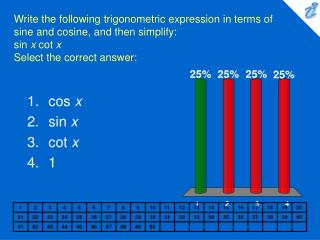DownloadDownload Presentationcos x sin x cot x 1

# cos x sin x cot x 1

Télécharger la présentation## cos x sin x cot x 1

- - - - - - - - - - - - - - - - - - - - - - - - - - - E N D - - - - - - - - - - - - - - - - - - - - - - - - - - -
##### Presentation Transcript

1. Write the following trigonometric expression in terms of sine and cosine, and then simplify: sin x cot xSelect the correct answer: • cos x • sin x • cot x • 1

2. Simplify the following trigonometric expression: {image} Select the correct answer: • sin 2x • sin x • sec 2x • 1

3. Simplify the following trigonometric expression as much as possible: {image} Select the correct answer: • cos 2x • sin 2x • sin x • csc x

4. Simplify the following trigonometric expression as much as possible: {image} Select the correct answer: • 2 sec x • sin x • 2 cos x • cos x

5. Simplify the following trigonometric expression as much as possible: {image} Select the correct answer: • 2 csc x • csc x • cot x • cot 2x